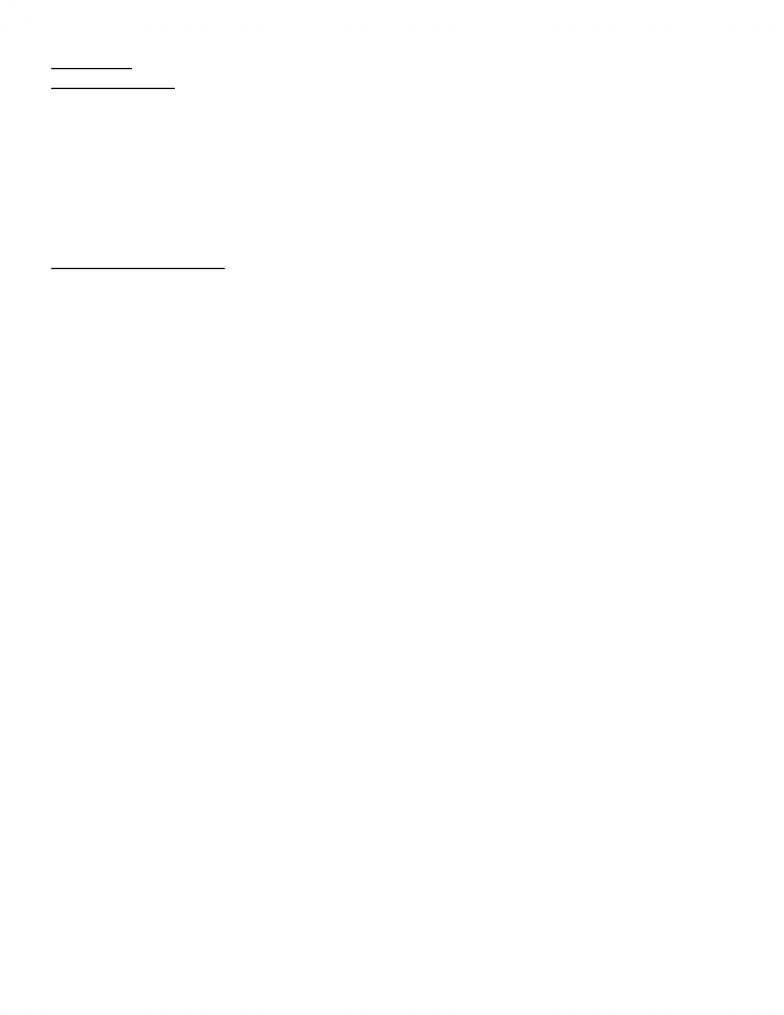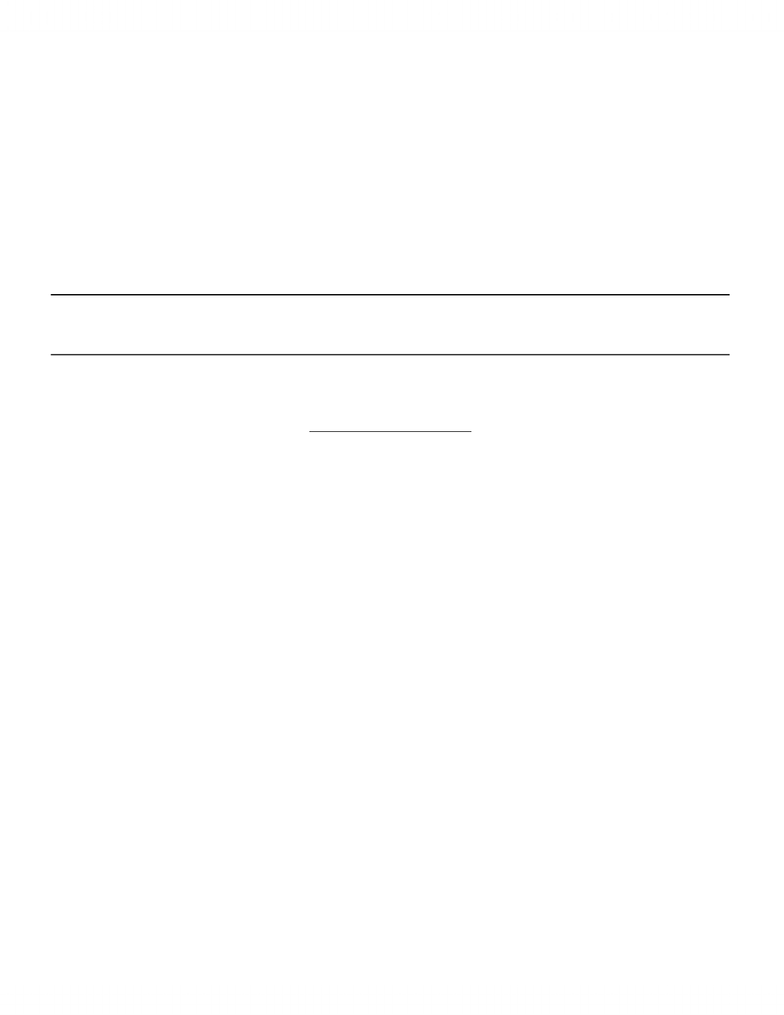# ECON 2GG3 Lecture Notes - Budget Constraint, Indifference Curve, Composite Good

79 views14 pagesChapter 3.
Demand Theory
Consumers face trade-offs in their decisions since incomes are limited and choices are
numerous. When you buy more of one good you can only afford less of the other.
In order to make choices, consumers combine
1. Preferences-what they would like to consume-We have analysed this in Chapter 2
2. Budget constraints-what they can afford.
The Budget Constraint
We are concern with the consumer's choice of the optimal bundle of goods and services.
Assumptions;
-- Income (M) is exogenous
--Prices are fixed and exogenous (p1 and p2 fixed).
--There is only 1 period (No savings nor bequests)
--Only consider two goods
Given these assumptions
Therefore total expenditure must be less than or equal to () income
or, p1x1 + p2x2 M.
Thus given income (M) possible combinations are determined by the prices of the two goods.
SEE FIG 3.1.
The equality divides the space into attainable and unattainable sets or bundles (given M and
pi’s).
The equality, known as the budget line, can be written as:
x2 = (M - p1x1)/p2
The slope is dx2/dx1 = - (p1/p2)
and the intercepts are: x2 = M/p2 (when x1 = 0)
and x1 =M/p1 (when x2 = 0)
See problem 3.1
(We will talk about endowments later)
What if M, p1, or p2 changes?
1
Unlock document

This preview shows pages 1-3 of the document.
Unlock all 14 pages and 3 million more documents.If M increases, how will the budget line shift/change?
If p1 increases, how will the budget line change?
If p2 decreases, how will the budget line change?
Next, consider the interior of the consumption set. Would it ever make sense to consume in
the interior of the budget line?
Non-satiation (more is better) implies that there is always a preferred bundle on the boundary
(budget line) – consider Fig 3.3
Therefore:
The non-satiation assumption/axiom implies that the utility-maximising consumption
bundle lies on the budget line.
The Choice Problem:
We can now state the problem formally (for the 2 goods case) as:
Maximize U = U(x1,x2) by choice of x1 and x2
subject to the constraint: p1x1 + p2x2 = M
One way of thinking of this is to find the highest indifference curve consistent with being in
the attainable set (on the budget constraint).
Figure 3.4 illustrates a solution (at point A)
Given the smoothness shown in the IC’s in Fig 3.4, it must be true at A, the highest IC
attainable:
1) you are on the budget line x2 = (M - p1x1)/p2
2) The slope of the IC = the slope of the Budget line.
i.e. - MU1/MU2 = - p1/p2
MRS=p1/p2
The solution (such as at point A) if written for x1 as:
x1* = f(M, p1,p2) is called the x1 demand function
For essential goods we will have an interior solution See figures 3.5a and 3.5b.
Inessetila goods See Fig. 3.6a and 36b
2
Unlock document

This preview shows pages 1-3 of the document.
Unlock all 14 pages and 3 million more documents.Fig. 3.6b-Here, given that at the vertical axis the budget line is steeper than the indifference
curve going through that same point, the optimal solution is on the vertical axis.
In this case at the optimum it is true that:
1) the individual is on the budget line
2) the budget line is steeper than the IC. Alternatively, we could say, MRS p1/p2.
3) the consumer spends all his/her income on x2 (x1 = 0).
The solution is given by the demand functions:
x1 = 0
x2 = M/p2
Note, this solution is common for a lot of goods you do not buy – eg helicopter rides,-In this
case good 1 is an inessential good. (Note that it is due to the high price of x1 that the
consumer doesn’t purchase it.
Note, alternatively, if it is everywhere true that the MRS is greater than the slope of the
budget line, then, the solution must be on the horizontal axis with x2 = 0!
Conclusion: even with all our axioms and assumptions, one can have an interior solution-
lies in the space between the two axes with MRS equal to the absolute value of the slope of
the budget line.
Or one can have a corner solution with either x1 or x2 equal to zero.
If a corner solution, then:
slope of IC flatter than BL means x1 = 0, x2 = M/p2
slope of IC steeper than BL means x2 = 0, x1 = M/p1
Example 1: Let p1 and p2 be unity so that the BC for an income of 100 becomes: 100 = x1 +
x2
Let U = x1x2
Find the solution to the Utility maximizing problem.
Problem could be stated as:
Maximize U=x1x2
Subject to
3
Unlock document

This preview shows pages 1-3 of the document.
Unlock all 14 pages and 3 million more documents.

## Document Summary

Consumers face trade-offs in their decisions since incomes are limited and choices are numerous. When you buy more of one good you can only afford less of the other. In order to make choices, consumers combine: preferences-what they would like to consume-we have analysed this in chapter 2, budget constraints-what they can afford. The budget constraint: we are concern with the consumer"s choice of the optimal bundle of goods and services. -prices are fixed and exogenous (p1 and p2 fixed). -there is only 1 period (no savings nor bequests) Therefore total expenditure must be less than or equal to ( ) income or, p1x1 + p2x2 . Thus given income (m) possible combinations are determined by the prices of the two goods. See fig 3. 1: the equality divides the space into attainable and unattainable sets or bundles (given m and. If p2 decreases, how will the budget line change: next, consider the interior of the consumption set.

# Get access

\$10 USD/m
Billed \$120 USD annually
Homework Help
Class Notes
Textbook Notes